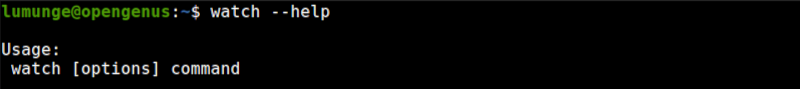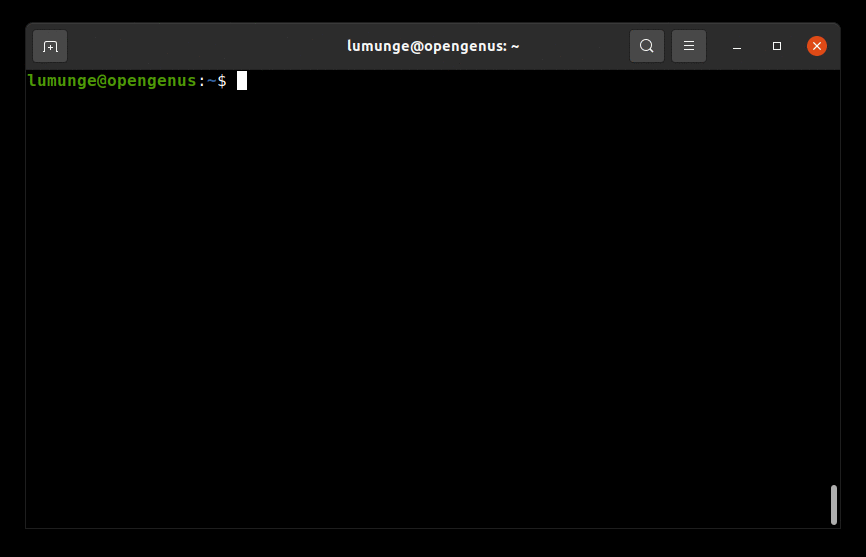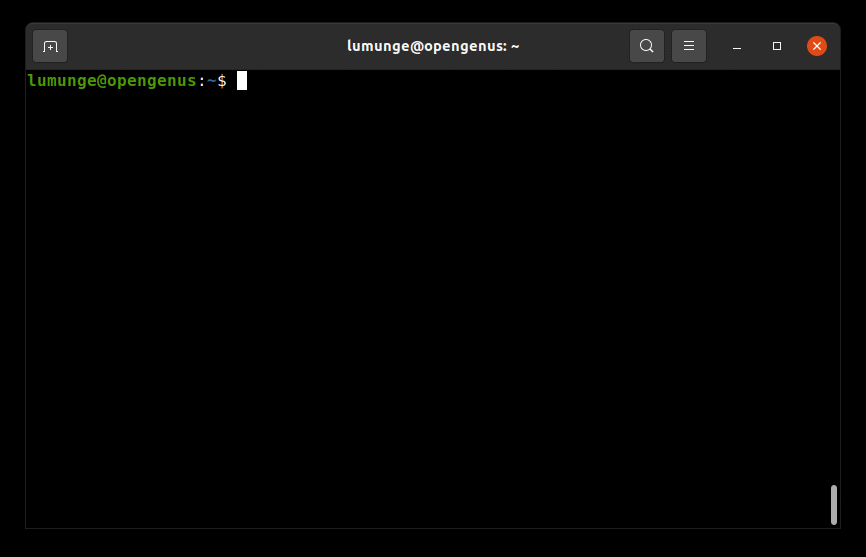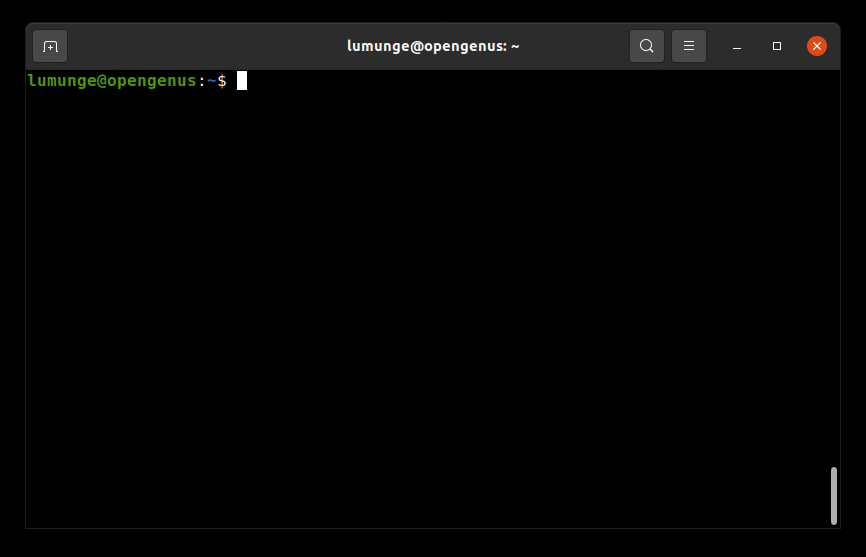×

Search anything:

# watch command in Linux

#### Linux Software EngineeringGet this book -> Problems on Array: For Interviews and Competitive Programming

We use the watch command to execute commands at regular intervals. We also use it for real-time logging of the command output. In this article, we discuss this command and understand it through various examples.

1. Introduction.
2. Syntax.
3. Command usage.
4. Summary.
5. References.

## Introduction.

We use the watch command to execute a command at regular intervals and print the output to the terminal.

This is useful in cases where we want to monitor real-time changes to a command's output. For example monitoring disk usage, firewall status, kernel activities, memory, cpu, and other system resources. We can also use it to monitor user activities in the system, files, data changes, etc.

## Syntax.

We write watch commands using the following syntax:

``````\$ watch [OPTIONS] [COMMAND]
``````## Command usage.

1. In Linux the date command is used to display the system's date and time. We can use the watch command with this command as follows:
``````\$ watch date
``````

Notice from the output that the date updates itself every 2 seconds.

1. We can change this interval by using the -n option followed by the specified number of seconds. For example, let's change the interval to every 5 seconds:
``````\$ watch -n 5 date
``````

The general syntax is as follows:

``````\$ watch -n [INTERVALS(SECS)] [COMMAND]
``````

Changing intervals of command execution is useful when the said command takes much of the system's resources. In this case, we need to prolong the intervals between updates.

1. To run the watch command at precise intervals, we use the -p option:
``````\$ watch -p date
``````
1. Looking at our date command example, between updates the number of seconds changes by a number of seconds, the default is two seconds but we can change this. These subtle changes can go unrecognized. We use the -d option to highlight differences between updates.
``````\$ watch -d date
``````Monitoring memory usage and spotting differences:

``````\$ watch -d free -m
``````1. Notice the header title from the output of the commands, We can eliminate it using the -t option:
``````\$ watch -t free -m
``````
1. If a command has an error, that is a non-zero exit status, we can exit it using the -e option:
``````\$ watch -e uptime
``````

In this example, if the uptime command has an error, the watch will not update the command's output, also when we press any key, the command will exit.

1. We can also opt to exit the watch command if the output changes. In this case, we use the -g option. For example, let's watch the date command, and if the output changes, we exit.
``````\$ watch -n 5 -g date
``````In this example, we set the interval to five seconds, that is, after five seconds the watch command will exit since we used the -g option.

After the command terminates, we can check its exit status. If the value is anything greater than 0, then there was an error. We can echo the exit status as follows:

``````\$ echo \$?
``````
1. We can also watch commands with pipes. For example, let's watch any changes made to a file by checking its file attributes listed using the ls command:
``````\$ watch -d 'ls -l | fgrep file.txt'
``````

After executing this command, we can try to add some content or whitespace. We will see its size changing in real-time.

1. To make the machine beep if a command exits with a non-zero exit, we use the -b option:
``````\$ watch -b 'll | fgrep file.txt
``````

## Summary.

In cases where we want to execute the same command repeatedly expecting a different result every time we use the watch command which logs real-time changes if any saving us a lot of time. Apart from logging we also saw how to make the watch command trigger a beep if a command exits with an error that notifies us.

For a comprehensive guide on the watch command, we can execute the command \$ man watch in a Linux terminal.

#### Erick Lumunge

Erick is a passionate programmer with a computer science background who loves to learn about and use code to impact lives positively.

watch command in Linux